Back to Contents Page

Lesson 4: Making objects with characteristics and behaviour with selection statements

This lesson will look at how we can use some of the commands available to us in Minecraft in order to make things respond to what we do in game. To do this we will make use of the selection statement that we learnt about previously, which is the 'if' statement.

First thing we will need is to familiarise our self with how to get the players position as we will be needing this a lot. The commands that we could use are:

mc.player.getPos()

and

mc.player.getTilePos()

Both functions return a set of 3D coordinates, the difference being that getPos() gives us the exact position of the player, whereas getTilePos() gives us the coordinates of the tile that the player is currently on. In order to get these coordinates into a usable form, we assign them to a variable in the following way:

x, y, z = mc.player.getPos() or x, y, z = mc.player.getTilePos()

Now we have the x, y and z coordinates saved into separate variables and we can use each independently.

So let's start with something simple, open up the game if you haven't already and create a new python file from the terminal to write your code into. At the top write in the following lines:

import mcpi.minecraft as minecraft

import mcpi.block as block

mc = minecraft.Minecraft.create()

Now, we want to check where the players position is, and then if they go more than 20 blocks in the x direction we will teleport them back to the start. We can set the players position using the following command:

mc.player.setPos(x, y, z)

Where the x, y and z are the coordinates that you choose to put the player at. So using these commands we can build the following statement:

x, y, z = mc.player.getPos()

if x > 20:

mc.player.setPos(0, 0, 0)

But if we run that now it will go through the code once and if the player is not already over 20 blocks away nothing will happen, to fix this we need to make the code repeat; we will do this using a while loop, with the exit condition set to True (what this does is mean that the loop will never stop).

You should end up with following code, save it and press f5 on the keyboard in order to run it.

import mcpi.minecraft as minecraft

import mcpi.block as block

mc = minecraft.Minecraft.create()

While True:

x, y, z = mc.player.getPos()

if x > 20:

mc.player.setPos(0, 0, 0)

Try walking more than +20 blocks in the x direction and test it out! When you want the code to stop running you need to click on the terminal and press 'ctrl' and 'c' together on your keyboard.

Challenge

That works in one direction, can you make it so it works for both directions? (Hint: your 'if' statement will need an 'or' in it somewhere)

Now see if you can do it for all 3 axes!

What happens if you dig a hole 20 blocks straight down from the start?

 Here’s where your position is shown

We can find your players current position by looking on the top left of the screen in Minecraft, using this you can go to a place you'd like to teleport to and remember the coordinates, try making a program containing the following code: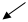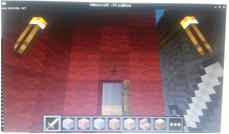import mcpi.minecraft as minecraft

import mcpi.block as block

mc = minecraft.Minecraft.create()

mc.player.setPos(x, y, z)

Where x, y and z are the coordinates that you found just now. Move away from that square and try running the code, you should teleport back to that square.

Challenge

Now try building a house and making a teleporter that makes you jump from one room to another!

For our final piece we are going to build a trap to keep those pesky intruders out! First we need to build a corridor leading to the front door of your house, it needs to be at least two blocks wide and 3 high. Next dig out the floor layer of all the blocks within the corridor.

Next we want to lay some stone blocks in a pattern in the pit that you dug so that you can jump from one block to another in order to get to the door of your house. Here is what a stone block looks like: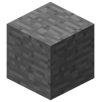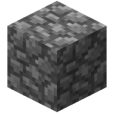Now that you've done that, you need to fill in any leftover spaces with some cobblestone blocks, they look like this:

You will end up with a floor that looks something like this: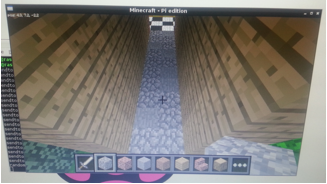From here we want to find the corner with the lowest x and z coordinates, so walk to each corner and look in the top left of the screen to find out which it is. Make a note of the two numbers and then head to the opposite corner which will have the highest x and z values, make a note of these too, and also the y coordinate value.

Now we want to create a statement that says if the player walks on a cobblestone square then make the floor disappear from beneath their feet. So to start with we are going to write:

x, y, z = mc.player.getTilePos()

if mc.getBlock(x, y, z) == 4:

mc.setBlocks(x, y, z, x, y – 20, z, 0)

So the command mc.getBlock(x, y, z) tells us what type of block there is at a given coordinate; it happens that '4' is the number that represents a cobblestone block. The next line sets the blocks from immediately below to 20 blocks below to block type '0' which is air.

To test your code is working go stand on a cobblestone block then try running the program, if all is well then youd should fall down a deep pit which has suddenly appeared. But once again this only happens when you run the program, we want it to keep checking if we have stood on a cobblestone block so we need to put it in a 'while True:' loop again!

While True:

x, y, z = mc.player.getTilePos()

if mc.getBlock(x, y, z) == 4:

mc.setBlocks(x, y, z, x, y – 20, z, 0)

Great, so that sort of does what we want, but now every single cobblestone block that we ever stand on is going to disappear when we stand on it; we only want that to happen in our corridor. That's where the numbers we wrote down earlier come in.

We need to create a statement to check that the player is within the corridor, so we write:

x, y, z = mc.player.getTilePos()

if a <= x <= b and c <= z <= d and y == e:

if mc.getBlock(x, y, z) == 4:

mc.setBlocks(x, y, z, x, y – 20, z, 0)

Where 'a' is the lower x number you wrote down, 'b' the upper x number, 'c' the lower z number and 'd' the upper z number; 'e' is the y value that you wrote. So putting it all together:

while True:

x, y, z = mc.player.getTilePos()

if a <= x <= b and c <= z <= d and y == e:

if mc.getBlock(x, y, z) == 4:

mc.setBlocks(x, y, z, x, y – 20, z, 0)

Now the only way to get safely through your corridor is to jump between the stone blocks!